Tamilnadu State Board New Syllabus Samacheer Kalvi 12th Computer Science Guide Pdf Chapter 16 Data Visualization Using Pyplot: Line Chart, Pie Chart and Bar Chart Text Book Back Questions and Answers, Notes.

Tamilnadu Samacheer Kalvi 12th Computer Science Solutions Chapter 16 Data Visualization Using Pyplot: Line Chart, Pie Chart and Bar Chart

12th Computer Science Guide Data Visualization Using Pyplot: Line Chart, Pie Chart and Bar Chart Text Book Questions and Answers

I. Choose the best answer (1 Mark)

Question 1.
Which is a python package used for 2D graphics?
a) matplotlib.pyplot
b) matplotlib.pip
c) matplotlib.numpy
d) matplotlib.plt
a) matplotlib.pyplotQuestion 2.
Identify the package manager for Python packages, or modules.
a) Matplotlib
b) PIP
c) plt.show()
d) python package
b) PIP

Question 3.
Read the following code: Identify the purpose of this code and choose the right option from the following.
C:\Users\YourName\AppData\Local\Programs\Python\Python36-32\Scripts > pip – version
a) Check if PIP is Installed
b) Install PIP
d) Check PIP version
d) Check PIP versionQuestion 4.
Read the following code: Identify the purpose of this code and choose the right option from the following.
a) List installed packages
b) list command
c) Install PIP
d) packages installed
a) List installed packages

Question 5.
To install matplotlib, the following function will be typed in your command prompt. What does “-U”represents?
Python -m pip install -U pip
c) removing pip

Question 6.
Observe the output figure. Identify the coding for obtaining this output.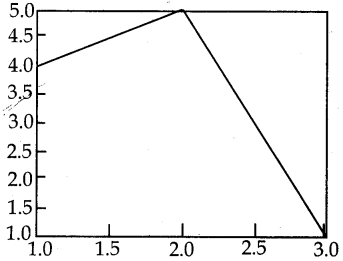a) import matplotlib.pyplot as pit
plt.plot([1,2,3],[4,5,1])
plt.show()

b) import matplotlib.pyplot as pit plt.
plot([1,2],[4,5])
plt.show()

c) import matplotlib.pyplot as pit
plt.plot([2,3],[5,1])
plt.showQ

d) import matplotlib.pyplot as pit pit .plot ([1,3], [4,1 ])
a) import matplotlib.pyplot as pit
plt.plot([1,2,3],[4,5,1])
plt.show()Question 7.
a. import matplotlib.pyplot as pit
b. plt.plot(3,2)
c. plt.show()
Identify the output for the above coding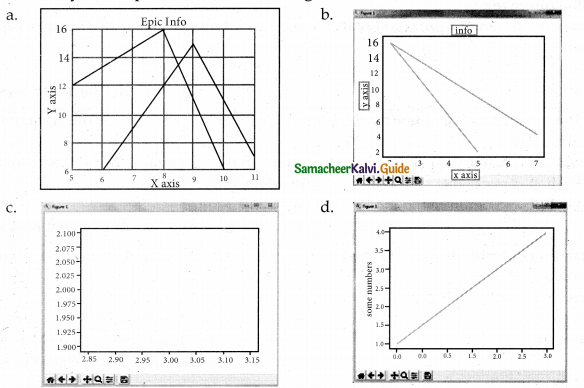c

Question 8.
Which key is used to run the module?
a) F6
b) F4
c) F3
d) F5
d) F5

Question 9.
Identify the right type of chart using the following hints.
Hint 1: This chart is often used to visualize a trend in data over intervals of time.
Hint 2: The line in this type of chart is often drawn chronologically.
a) Line chart
b) Bar chart
c) Pie chart
d) Scatter plot
a) Line chartQuestion 10.
Read the statements given below. Identify the right option from the following for pie chart. Statement A : To make a pie chart with Matplotlib, we can use the plt.pie() function.
Statement B : The autopct parameter allows us to display the percentage value using the Python string formatting.
a) Statement A is correct
b) Statement B is correct
c) Both the statements are correct
d) Both the statements are wrong
b) Statement B is correct

II Answer the following questions (2 marks)

Question 1
Define Data Visualization.
Data Visualization is the graphical representation of information and data. The objective of Data Visualization is to communicate information visually to users. For this, data visualization uses statistical graphics. Numerical data may be encoded using dots, lines, or bars, to visually communicate a quantitative message.

Question 2.
List the general types of data visualization.

• Charts
• Tables
• Graphs
• Maps
• Infographics
• Dashboards

Question 3.
List the types of Visualizations in Matplotlib.
There are many types of Visualizations under Matplotlib. Some of them are:

1. Line plot
2. Scatter plot
3. Histogram
4. Box plot
5. Bar chart and
6. Pie chartQuestion 4.
How will you install Matplotlib?

• Pip is a management software for installing python packages.
• We can install matplotlib using pip.
• First of all, we need to identify whether pip is installed in your PC. If so, upgrade the pip in your system.
• To check if pip is already installed in our system, navigate our command line to the location of Python’s script directory.
• You can install the latest version of pip from our command prompt using the following command:
Python -m pip install -U pip
• To install matplotlib, type the following in our command prompt:
Python -m pip install -U matplotlib

Question 5.
Write the difference between the following functions: plt. plot([1,2,3,4]), plt. plot ([1,2,3,4], [14, 9,16]).

 plt.plot([I,2,3,4]) plt.pIot([I,2,3,4],[1,4,9,16]) 1 In plt.plot([1,2,3,4]) a single list or array is provided to the plot() command. In plt.pIot([1,2,3,4],[l,4,9,16]) the first two parameters are ‘x’ and ‘y’ coordinates. 2 matplotlib assumes it is a sequence of y values, and automatically generates the x values. This means, we have 4 co-ordinate according to these ‘list as (1,1), (2,4), (3,9) and (4,16). 3 Since python ranges start with 0, the default x vector has the same length as y but starts with 0. Hence the x data are [0,1,2,3].

III. Answer the following questions (3 marks)

Question 1.
Draw the output for the following data visualization plot.
import matplotlib.pyplot as plt
plt.bar([1,3,5,7,9],[5,2,7,8,2], label=”Example one”)
plt.bar([2,4,6,8,10],[8,6,2,5,6], label=:”Example two”, color=’g’)
plt.legend()
plt.xlabel(‘bar number’)
plt.ylabel(‘bar height’)
plt.title(‘Epic Graph\nAnother Line! Whoa’)
plt.show()
Output: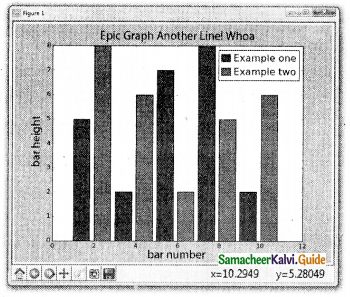Question 2.
Write any three uses of data visualization.

1. Data Visualization helps users to analyze and interpret the data easily.
2. It makes complex data understandable and usable.
3. Various Charts in Data Visualization helps to show the relationship in the data for one or more variables.Question 3.
Write the coding for the following:
a) To check if PIP is Installed in your PC.
b) To Check the version of PIP installed in your PC.
c) To list the packages in matplotlib.
a) To check if PIP is Installed in your PC.

1. In command prompt type pip – version.
2. If it is installed already, you will get version.
3. Command : Python – m pip install – U pip

b) To Check the version of PIP installed in your PC.
C:\ Users\ YourName\ AppData\ Local\ Programs\ Py thon\ Py thon36-32\ Scripts>
pip-version

c) To list the packages in matplotlib.
C:\ Users\ Y ou rName\ AppData\ Local\ Programs\ Py thon\ Py thon36-32\ Scripts>
pip list

Question 4.
Write the plot for the following pie chart output.
Coding:
import matplotlib. pyplot as pit
sizes = [54.2,8.3,8.3,29.2]
labels= [“playing”,”working”,”eating”,”sleeping”]
exp=(0,0,0.1,0)
pit.pie (sizes labels = labels,explode=exp,autopct=”%.If%%, shadow-True, counterclock=False,strartangle-90)
pit, axes (). set_aspect (“equal”)
pit. title (“Interesting Graph \nCheck it out”)
plt.show ()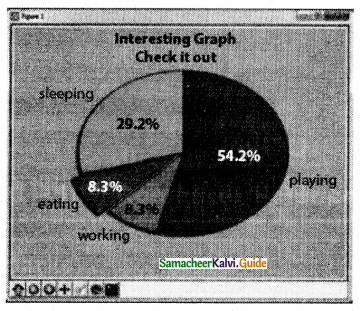IV. Answer the following questions (5 Marks)

Question 1.
Explain in detail the types of pyplots using Matplotlib.
Matplotlib allows us to create different kinds of plots ranging from histograms and scatter plots to bar graphs and bar charts.

Line Chart:

• A Line Chart or Line Graph is a type of chart which displays information as a series of data points called ‘markers’ connected by straight line segments.
• A Line Chart is often used to visualize a trend in data over intervals of time – a time series – thus the line is often drawn chronologically.

Program for Line plot:
import matplotlib.pyplot as pit years = [2014, 2015, 2016, 2017, 2018] total_populations = [8939007, 8954518,, 8960387,8956741,8943721]
plt. plot (“years, total_populations)
plt. title (“Year vs Population in India”)
plt.xlabel (“Year”)
plt.ylabel (“Total Population”)
plt.showt

In this program,
Plt.titlet() →→7 specifies title to the graph
Plt.xlabelt) →→ specifies label for X-axis
Plt.ylabelO →→ specifies label for Y-axis
Output: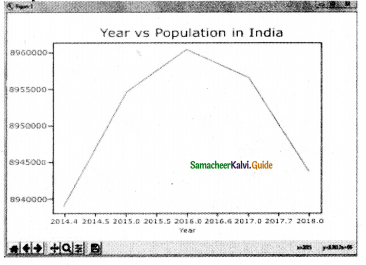Bar Chart:

• A BarPlot (or Bar Chart) is one of. the most common type of plot. It shows the relationship between a numerical variable and a categorical variable.
• Bar chart represents categorical data with rectangular bars. Each bar has a height corresponds to the value it represents.
• The bars can be plotted vertically or horizontally.
• It is useful when we want to compare a given numeric value on different categories.
• To make a bar chart with Matplotlib, we can use the plt.bart) function.

Program:
import matplotlib.pyplot as pit
# Our data
labels = [“TAMIL”, “ENGLISH”, “MATHS”, “PHYSICS”, “CHEMISTRY”, “CS”]
usage = [79.8,67.3,77.8,68.4,70.2,88.5]
# Generating the y positions. Later, we’ll use them to replace them with labels. y_positions = range (len(labels))
# Creating our bar plot
plt.bar (y_positions, usage)
plt.xticks (y_positions, labels)
plt.ylabel (“RANGE”)
pit.title (“MARKS”)
plt.show()
Output: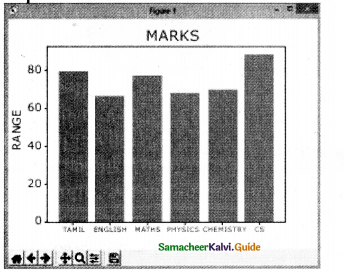Pie Chart:

• Pie Chart is probably one of the most common type of chart.
• It is a circular graphic which is divided into slices to illustrate numerical proportion.
• The point of a pie chart is to show the relationship of parts out of a whole.
• To make a Pie Chart with Matplotlib, we can use the plt.pie () function.
• The autopct parameter allows us to display the percentage value using the Python string formatting.

Program:
import matplotlib.pyplot as pit
sizes = [89, 80, 90,100, 75]
labels = [“Tamil”, “English”, “Maths”,
“Science”, “Social”]
plt.pie (sizes, labels = labels,
autopct = “%.2f”)
plt.axesfj.set aspect (“equal”)
plt.showt()
Output: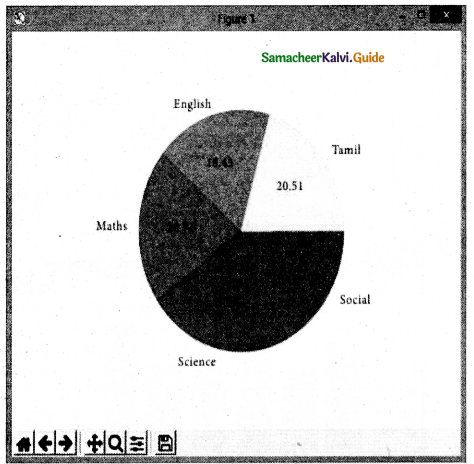Question 2.
Explain the various buttons in a matplotlib window.
Various buttons in a matplotlib window: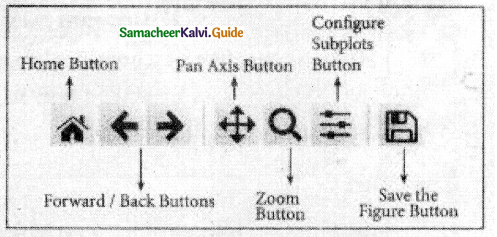Home Button → The Home Button will help once you have begun navigating your chart. If you ever want to return back to the original view, you can click on this.
Forward/Back buttons → These buttons can be used like the Forward and Back buttons in your browser. You can click these to move back to the previous point you were at, or forward again.
Pan Axis → This cross-looking button allows you to click it, and then click and drag your graph around.
Zoom → The Zoom button lets you click on it, then click and drag a square that you would like to zoom into specifically. Zooming in will require a left click and drag. You can alternatively zoom out with a right click and drag.
Configure Subplots → This button allows you to configure various spacing options with your figure and plot.
Save Figure → This button will allow you to save your figure in various forms.

Question 3.
Explain the purpose of the following functions:
a) plt.xlabel:
plt.xlabel Specifies label for X -axis
b) plt.ylabel:
plt.ylabel is used to specify label for y-axis
c) plt.title :
plt.title is used to specify title to the graph or assigns the plot title.
d) plt.legend():
plt.legend() is used to invoke the default legend with plt
e) plt.show():
plhshowQ is used to display the plot.12th Computer Science Guide Data Visualization Using Pyplot: Line Chart, Pie Chart and Bar Chart Additional Questions and Answers

I. Choose the best answer (1 Mark)

Question 1.
………………. button is used to click and drag a graph around.
a) pan axis
b) home
c) zoom
d) drag
a) pan axis

Question 2.
………………. charts display information as series of data points.
a) Bar
b) Pie
c) Line
d) Histogram
c) LineQuestion 3.
The representation of information in a graphic format is called …………………………
(a) chart
(b) graphics
(c) Infographics
(d) graphs
c) Infographics

Question 4.
……………….refers to a graphical representation that displays data by way of bars to show the frequency of numerical data.
a) Bar chart
b) Line graph
c) Pie chart
d) Histogram
d) Histogram

Question 5.
……………….represents the frequency distribution of continuous variables.
a) Bar chart
b) Line graph
c) Pie chart
d) Histogram
d) Histogram

Question 6.
Find the Incorrect match from the following.
(a) Scatter plot – collection of points
(b) line charts – markers
(c) Box plot – Boxes
(c) Box plot – BoxesQuestion 7.
Which of the following plot we cannot rearrange the blocks from highest to lowest?
a) Line
b) Bar chart
c) Pie chart
d) Histogram
d) Histogram

Question 8.
In ………………. graph, the width of the bars is always the same.
a) Line
b) Bar
c) Pie chart
d) Histogram
b) Bar

Question 9.
The ………………. parameter allows us to display the percentage value using the Python string formatting in pie chart.
a) percent
b) autopct
c) pet
d) percentage
b) autopct

Question 10.
Find the wrong statement.
If a single list is given to the plot( ) command, matplotlib assumes
(a) it is as a sequence of x values
(b) the sequence of y values
(a) it is as a sequence of x valuesQuestion 11.
………………. is the graphical representation of information and data.
a) Data visualization
b) Data Graphics
c) Data Dimension
d) Data Images
a) Data visualization

Question 12.
………………. in data visualization helps to show the relationship in the data for more variables.
a) Tables
b) Graphics
c) Charts
d) Dashboards
c) Charts

Question 13.
In a Scatter plot, the position of a point depends on its …………………………. value where each value is a position on either the horizontal or vertical dimension.
a) 2-Dimensional
b) 3-Dimensional
c) Single Dimensional
d) 4-Dimensional
a) 2-Dimensional

Question 14.
……………………. plot shows the relationship between a numerical variable and a categorical variable.
(a) line
(b) Bar
(c) Scatter
(d) Box
(b) BarQuestion 15.
…………………. is the representation of information in a graphic format.
a) Infographics
b) Graph
c) Symbol
d) Charts
a) Infographics

Question 16.
…………………. is a collection of resources assembled to create a single unified visual display.
a) Infographics
b) Dashboard
c) Graph
d) Charts
b) Dashboard

Question 17.
Matplotlib is a data visualization …………………. in Python.
a) control structure
b) dictionary
c) library
d) list
c) library

Question 18.
Matplotlib allows us to create different kinds of …………………. ranging from histograms
a) Table
b) Charts
c) Maps
d) plots
d) plotsQuestion 19.
Which function shows the percentage value in the pie chart?
(a) percent
(b) percentage
(c) slice
(d) auto pet
(d) auto pet

Question 20.
…………………. command will take an arbitrary number of arguments.
a) show ()
b) plot ()
c) legend ()
d) title ()
b) plot ()

Question 21.
The most popular data visualization library allows creating charts in few lines of code in python.
a) Molplotlib
b) Infographics
c) Data visualization
d) pip
a) MolplotlibII. Answer the following questions (2 and 3 Marks)

Question 1.
What is meant by Infographics?
Infographics → An infographic (information graphic) is the representation of information in a graphic format.

Question 2.
Define Dashboard.

• A dashboard is a collection of resources assembled to create a single unified visual display.
• Data visualizations and dashboards translate complex ideas and concepts into a simple visual format.
• Patterns and relationships that are undetectable in the text are detectable at a glance using the dashboard.

Question 3.
Write a note on matplotlib

• Matplotlib is the most popular data visualization library in Python.
• It allows creating charts in few lines of code.

Question 4.
Write a note on the scatter plot.

• A scatter plot is a type of plot that shows the data as a collection of points.
• The position of a point depends on its two dimensional value, where each value is a position on either the horizontal or vertical dimension.

Question 3.
What is Box Plot?
Box plot: The box plot is a standardized way of displaying the distribution of data based on the five-number summary: minimum, first quartile, median, third quartile, and maximum.III. Answer the following questions (5 Marks)

Question 1.
Write the key differences between Histogram and bar graph.

 Histogram Bar graph 1 Histogram refers to a graphical representation; that displays data by way of bars to show the frequency of numerical data. A bar graph is a pictorial representation of data that uses bars to compare different categories of data. 2 A histogram represents the frequency distribution of continuous variables. A bar graph is a diagrammatic comparison of discrete variables. 3 The histogram presents numerical data The bar graph shows categorical data 4 The histogram is drawn in such a way that there is no gap between the bars. There is proper spacing between bars in a bar graph that indicates discontinuity. 5 Items of the histogram are numbers, which are categorised together, to represent ranges of data. Items are considered as individual entities. 6 A histogram, rearranging the blocks, from highest to lowest cannot be done, as they are shown in the sequence of classes. In the case of a bar graph, it is quite common to rearrange the blocks, from highest to lowest. 7 The width of rectangular blocks in a histogram may or may not be the same. The width of the bars in a bar graph is always the same.

Question 2.
Explain the purpose of
i) plt.plot()
ii) pt.bar()
iii) plt.sticks()
iv) plt.pie
i) plt.plot():
plt.plot() is used to make a line chart or graph with matplotlib.

ii) plt.bar():
plt.bar() is used to make a bar chart with matplotlib.

iii) plt.xticks():

• plt.xticks() display the tick marks along the x-axis at the values represented.
• Then specify the label for each tick mark.
• It is used bar chart.

iv) plt.pie ():
plt.pie () is used to make a pie chart with matplotlib.

Question 3.
Draw the output for the following python code:
import matplotlib.pyplot as pit
a = [1,2,3]
b = [5,7,4]
x = [1,2,3]
y = [10,14,12]
plt.plot(a,b, label=/Lable 1′)
plt.plot(x,y, label=’Lable 2′)
plt.xlabel(‘X-Axis’)
plt.ylabel(‘Y-Axis’)
plt. legend ()
plt. show ()
Output: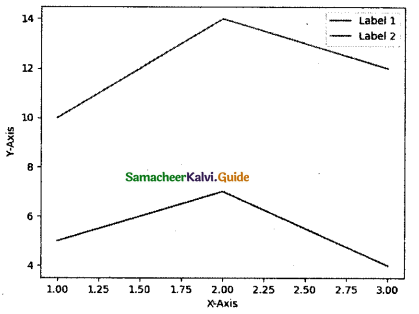Question 4.
Draw the chart for the given Python snippet.
import matplotlib.pyplot as plt
plt.plot([l,2,3,4], [1,4,9,16])
plt.show()
Output: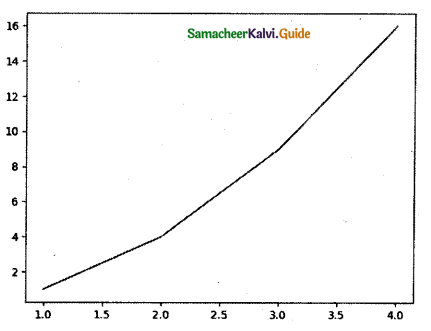Hands-on Practice

Question 1.
Create a plot. Set the title, the x and y labels for both axes,
import matplotlib.pyplot as pit
x=[1,2,3]
Y=[5,7,4]
plt.plot(x,y)
plt.xlable(‘X-AXIS’)
plt.ylabel(‘Y -AXIS’)
plt.title(‘LINE GRAPH)
plt.show()

Question 2.
Plot a pie chart for your marks in the recent examination.
import matplotlib.pyplot as pit
s=[60,85,90,83,95] l=[‘LANG’,’ENG’,’MAT ‘,’SCI’,’SS’]
plt.pie(s,labels=l)
plt.title(‘MARKS’)
plt.show()Question 3.
Plot a line chart on the academic performance of Class 12 students in Computer Science for the past 10 years.
import matplotlib.pyplot as pit
x=[2009,2010,2011,2012,2013,2014,2015,20 16,2017,2018]
y=[56,68,97,88,92,96,98,99,100,100]
plt.plot(x,y) plt.xlable(‘YEAR’)
plt.ylabel(‘PASS % IN C.S’)
plt. show ()

Question 4.
Plot a bar chart for the number of computer science periods in a week, import matplotlib.pyplot as pit x=[“MON”,”TUE”,”WED”, “THUR”,”FRI”]
y=[6,5,2,1,7] plt.bar(x,y) pit. xlable (‘ DAY S’) plt.ylabel(‘PERIOD’) plt.showQ